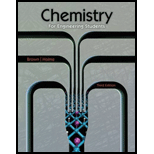# 4.50 Aluminum hydroxide can be dissolved in hydrochloric acid to form aluminum chloride and water. Write a balanced chemical equation for this reaction. How many mL of 3.37 M HCI is needed to react with 10.0 g of Al(OH) 3 ?### Chemistry for Engineering Students

3rd Edition
Lawrence S. Brown + 1 other
Publisher: Cengage Learning
ISBN: 9781285199023

#### Solutions### Chemistry for Engineering Students

3rd Edition
Lawrence S. Brown + 1 other
Publisher: Cengage Learning
ISBN: 9781285199023
Chapter 4, Problem 4.52PAE
Textbook Problem
185 views

## 4.50 Aluminum hydroxide can be dissolved in hydrochloric acid to form aluminum chloride and water. Write a balanced chemical equation for this reaction. How many mL of 3.37 M HCI is needed to react with 10.0 g of Al(OH)3?

Interpretation Introduction

Interpretation:

To write a chemical equation and calculate the volume of HCl required.

Concept introduction:

• The maximum yield is obtained by reacting all the limiting reactant.
• Percent yield relates actual versus theoretical yields.
• Molarity measures molar concentration.

Given:

Molarity =3.37MHClmass =10.0gAl(OH)3Molecular Weight Al(OH)3=78.0 g/mol

### Explanation of Solution

When performing calculations with molar concentration (molarity) it is much easier to change all units to moles.

Step 1: Balance Reaction

HCl(aq)+Al(OH)3(aq)H2O(l)+AlCl3(aq)3HCl(aq)+Al(OH)3(aq)3H2O(l)+AlCl3(aq)

Reaction is balanced

Step 2: Change all amounts to mol

molAl(OH)3=massMolecular Weight=10.078.0=0.1282 mole

Step 3: Relate stoichiometry

Ratio=1:3

molHCl=3×(molAl(OH)3=3×0

### Still sussing out bartleby?

Check out a sample textbook solution.

See a sample solution

#### The Solution to Your Study Problems

Bartleby provides explanations to thousands of textbook problems written by our experts, many with advanced degrees!

Get Started

Find more solutions based on key concepts
What molecular formula corresponds to each of the following structural formulas?

General Chemistry - Standalone book (MindTap Course List)

Organic nutrients include all of the following except. a. minerals b. fat c. carbohydrates d. protein

Nutrition: Concepts and Controversies - Standalone book (MindTap Course List)

What is triacylglycerol mobilization?

Organic And Biological Chemistry

The distance between the centers of the wheels of a motorcycle is 155 cm. The center of mass of the motorcycle,...

Physics for Scientists and Engineers, Technology Update (No access codes included)